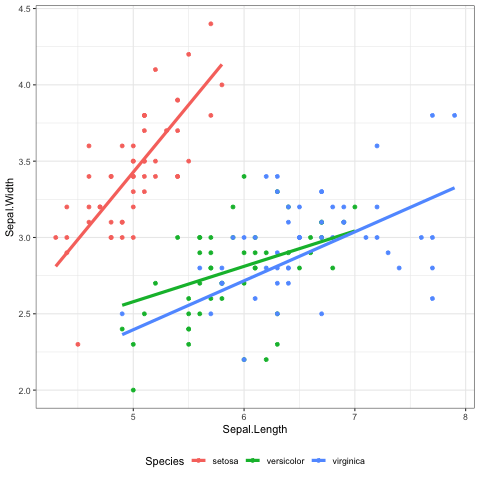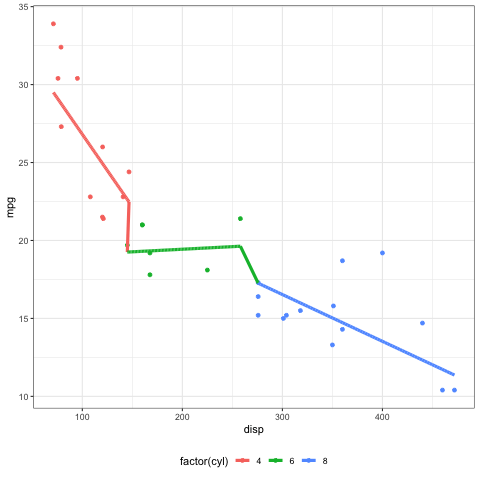{{ message }}

# Strange dataset dependent grouping effect#266

Closed
opened this issue Jan 7, 2019 · 3 comments
Closed

# Strange dataset dependent grouping effect#266

opened this issue Jan 7, 2019 · 3 comments

###clauswilke commented Jan 7, 2019

 This works: ```library(ggplot2) library(ungeviz) # devtools::install_github("wilkelab/ungeviz") library(gganimate) ggplot(iris, aes(Sepal.Length, Sepal.Width, colour = Species)) + geom_point() + stat_smooth_draws( times = 10, formula = y ~ x, aes(group = interaction(stat(.draw), colour)), size = 1.5 ) + theme_bw() + theme(legend.position = "bottom") + transition_states(stat(.draw), 1, 2) + enter_fade() + exit_fade()```This doesn't (lines are now connected): ```ggplot(mtcars, aes(disp, mpg, colour = factor(cyl))) + geom_point() + stat_smooth_draws( times = 10, formula = y ~ x, aes(group = interaction(stat(.draw), colour)), size = 1.5 ) + theme_bw() + theme(legend.position = "bottom") + transition_states(stat(.draw), 1, 2) + enter_fade() + exit_fade()```Notice that the only difference is the data set. I have tried `as.character(cyl)` instead of `factor(cyl)` to see if the problem is the numeric variable turned into a factor, and it makes no difference. The text was updated successfully, but these errors were encountered:

###thomasp85 commented Jan 7, 2019

 At least you can't complain the fixes aren't coming fairly quickly :-)

###clauswilke commented Jan 7, 2019

 I'm not complaining. Thanks for all your effort on this. `shadow_mark()` looks much better now with `geom_smooth()`!

###thomasp85 commented Jan 7, 2019

 Dont worry - just joking 🙃